Author and Editorialist: Simon St James
Tester: Suchan Park

# DIFFICULTY:

Medium-Hard TODO - revisit this - seems harder than MVCN2TST

# PREREQUISITES:

Sprague-Grundy, Centroid Decomposition, Bit Manipulation, Ad-hoc

# PROBLEM:

Bob and Alice are playing a game on a board which is a tree, $T$. Each node of $T$ has some number of coins placed on it. For a node $R$, define $\textit{game}(T, R)$ to be the game played on $T$ in which players take turns to move a coin from some node other than $R$ strictly towards $R$. The first player unable to make a move loses the game. For each $R$, find the winner of $game(T,R)$, assuming both players play perfectly.

# QUICK EXPLANATION:

The game $\textit{game}(T, R)$ is equivalent to the game of Nim, where there is a one-to-one correspondence between coins on the board and piles of stones: for a coin $c$, if $v_c$ is the node containing $c$, then $c$ corresponds to a Nim pile of size $\textit{dist}(R,v_c)$. Thus, the Sprague-Grundy Theorem can be used. Some simple observations show that the exact value of $c_v$ is not important; only its parity: we set $V_{\textit{coin}}$ to be the set of nodes $v$ such that $c_v$ is odd.

Define

$R.\textit{grundy}=\bigoplus_{v\in V_{\textit{coin}}}{\textit{dist}(R,v)}$

Then by the Sprague-Grundy Theorem, the second player (Bob) wins $\textit{game}(T, R)$ if and only if $R.\textit{grundy}=0$, so we need only calculate this value for all $R$.

For nodes $u$ and $v$ where $v\in V_{\textit{coin}}$, define the contribution of $v$ to $u$ as $\textit{dist}(u,v)$, and the act of updating $u.\textit{grundy}=u.\textit{grundy}\oplus \textit{dist}(u,v)$ as propagating $v$'s contribution to $u$.

We form a $\textit{DistTracker}$ data structure with the following API:

class DistTracker
insertDist(distance) { ... }
grundyNumber() { ... } // Return the xor sum of all the contained distances 

In a naive implementation, at least one of these operations would be $\mathcal{O}(N)$, but by observing how bits in the binary representation of a number change upon incrementing it and using some properties of xor, we can implement all of $\textit{DistTracker}$'s operations in $\mathcal{O}(\log N)$ or better.

We then use Centroid Decomposition plus our $\textit{DistTracker}$ to collect all contributions of $v$ with $v\in V_{\textit{coin}}$ and propagate them to all nodes $R$, thus calculating all required $R.\textit{grundy}$.

# EXPLANATION:

As mentioned, this is the game of Nim in disguise: in Nim, we start with some number $M$ of piles, the $i^\textit{th}$ of which contains $p_i$ stones, and players take turns to choose a non-empty pile and take at least one stone from it, until a player cannot make a move, in which case they lose. In the game $\textit{game}(T, R)$, let $v_C$ be the node containing the coin $C$; then the correspondence between the two games is as follows:

• Each coin $c$ Corresponds to a pile of size $\textit{dist}(R,v_C)$ in the game of Nim
• Choosing a coin $C$ from a node not in $R$ and moving it strictly towards $R$ corresponds to choosing a non-empty pile of stones and taking at least one stone from it
• The losing state - where all coins are in $R$ - corresponds to the losing state in Nim where all piles are empty.

The Sprague-Grundy Theorem proves several interesting statements but the one we're interested in is the remarkable (and very unintuitive!) result that in the game of Nim, the second player wins if and only if the Grundy Number for the game is $0$, where the grundy number is the xor-sum of all the pile sizes i.e. $p_1 \oplus p_2 \oplus \dots \oplus p_M$. Applying this to our game, we see that Bob wins if and only if the grundy number $R.\textit{grundy}$ for the game $\textit{game}(T, R)$ defined by:

$R.\textit{grundy}=\bigoplus_{c\in \textit{coins}}{\textit{dist}(R,v_c)}$

is $0$.

We can simplify this a little: consider two coins $C_A$ and $C_B$, both on the same node $v$. Their contribution to $R.\textit{grundy}$ is $\textit{dist}(R, v) \oplus \textit{dist}(R, v)$. But $x \oplus x = 0$ for all $x$, so we can remove both coins without affecting $R.\textit{grundy}$. For each $v$, we can safely remove pairs of coins from $v$ until either $0$ or $1$ remain, depending on whether $c_v$ was originally odd or even. We say that a node $v$ $\textit{hasCoin}$ if $c_v$ is odd, and set $V_\textit{coin}=$ the set of all such $v$. We can now rephrase the formula for $R.\textit{grundy}$:

$R.\textit{grundy}=\bigoplus_{v \in V_{\textit{coin}} }{\textit{dist}(R,v)}$

Recalling the definitions of contribution and propagation from the Quick Explanation, we see that to solve the problem, we merely need to ensure that for each $v \in V_{\textit{coin}}$ and every $R$, $v$'s contribution to $R$ is propagated to $R$.

Let's consider for the moment the special case where $T$ is simply a long chain of nodes. Imagine we had a $\textit{DistTracker}$ data structure with the below API (a naive implementation is also provided):

    class DistTracker
public:
insertDist(distance)
{
trackedDistances.append(distance)
}
{
for each distance in trackedDistances:
}
grundyNumber()
{
result = 0
for each distance in trackedDistances:
result = result ^ distance
return result
}
clear()
{
trackedDistances = []
}
private:
trackedDistances = []


Imagine further that we proceed along the chain of nodes from left to right performing at each node $v$ the following steps:

• Propagate all the contributions of nodes that $\textit{hasCoin}$ we've seen so far to $v$ via $v.grundy=v.grundy \oplus \textit{distTracker}.\textit{grundyNumber}()$
• If $v\in V_{\textit{coin}}$, collect the contribution of $v$ via $\textit{distTracker}.\textit{insertDist}(0)$
• Move to the next node in the chain, calling $\textit{distTracker}.\textit{addToAllDists}(1)$ to update the contributions we've collected as we go

(Click image to see the animation)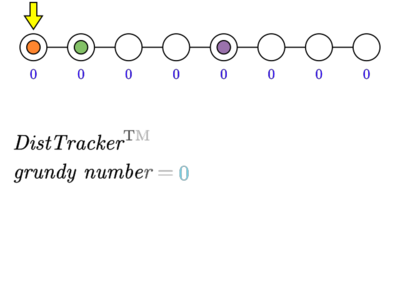This way, we collect then propagate the contribution of each $v\in V_{\textit{coin}}$ to all nodes to $v$'s right.

Let's $\textit{clear}()$ our $\textit{distTracker}$ and repeat the process, this time working in the opposite direction: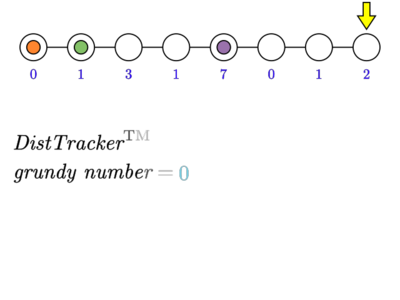Now we've propagated the contribution of each $v \in V_{\textit{coin}}$ to all nodes to $v$'s right and to its left i.e. to all nodes, and so have computed all $R.\textit{grundy}$, as required. It turns out that Bob wins two of the games and Alice wins the rest.

The naive implementation of $\textit{DistTracker}$ given above is too slow to be of use: we'll fix this later but first, let's show how we can use Centroid Decomposition with our $\textit{DistTracker}$ to collect and propagate all $v\in V_{coin}$'s on an arbitrary tree $T$. If you're already familiar with Centroid Decomposition, you may want to skip this part.

Using Centroid Decomposition to Propagate All Contributions We won't go into much detail on Centroid Decomposition here as there are many resources about it, but here are the properties we care about:

C1: Centroid Decomposition of $T$ induces $M$ subtrees ($M$ is $\mathcal{O}(N)$) $T_i$ of $T$ each with a node $C_i$ that is the centre of $T_i$
C2: The $\Sigma_{i=1}^M |T_i|$ is $\mathcal{O}(N\log N)$
C3: Let $u,v$ be any distinct pair of nodes, and let $P(u,v)=[u=p_0, p_1, \ldots, p_k=v]$ be the unique path between $u$ and $v$. Then there is precisely one $i$ such that $u$ and $v$ are in subtree $T_i$ and $c_i \in P(u,v)$

Let $D_i$ be the degree of $C_i$ in $T_i$, and let $b_1, b_2, \dots, b_{D_i}$ be the neighbours of $C_i$ in $T_i$. We partition the $u\in T_i$, $u \ne C_i$ into $D_i$ branches, where the node $u$ is in branch $l$ if the unique path from $C_i$ to $u$ passes through $b_l$. For example:

TODO - image here - medium size $T_i$ with $D_i = 4$ - MOVCOIN2_ED_3_THUMB.png, linking to MOVCOIN2_ED_3_ANIM.gif. In the meantime, you can probably figure it out from anims 6 & 7 XD

With this notation, C3 can be rephrased as:

C3: Let $u,v$ be any distinct pair of nodes; then there is precisely one $i$ such that $u$ and $v$ are in subtree $T_i$ and either:

1. $C_i=u$; or
2. $C_i=v$; or
3. $u$ and $v$ are in different branches of $T_i$

from which it follows that doing the following for every $i=1,2,\ldots, M$:

1. for each $j=1,2,\dots,D_i$, propagate the contributions of all $v \in V_\textit{coin}\cap B_j$ to the nodes in the other $D_i-1$ branches of $T_i$; and
2. propagate the contributions of all $v \in V_\textit{coin}\cap T_i$ to $C_i$; and
3. (if $C_i.\textit{hasCoin}$) propagate the contribution of $C_i$ to all other nodes in $T_i$

will propagate the contributions of all $v \in V_{\textit{coin}}$ to all $u \in T$, as required.

Both 1. and 2. can be done separately using a naive algorithm (although my implementation rolls them into 3.). 3. can be handled in a similar way to the "propagate-and-collect-and-then-in-reverse" approach from earlier, except now we are collecting and propagating branches at a time, rather than nodes.

For each $i=1,2,\dots,M$, create a fresh $\textit{DistTracker}$ and perform the following steps:

1. Propagate the contributions to nodes in branch $i$; that is, do a DFS from $b_i$, calling $\textit{addToAllDists}(1)$ when we visit a node for the first time, and $\textit{addToAllDists}(-1)$ when we have fully explored it
2. Collect the contributions of nodes in branch $i$; that is, do a DFS from $b_i$, calling $\textit{insertDist}(d)$ when we encounter a node in $V_{\textit{coin}}$ at distance $d$ from $C_i$.

A BFS would also work and would likely be slightly more efficient: here's an example: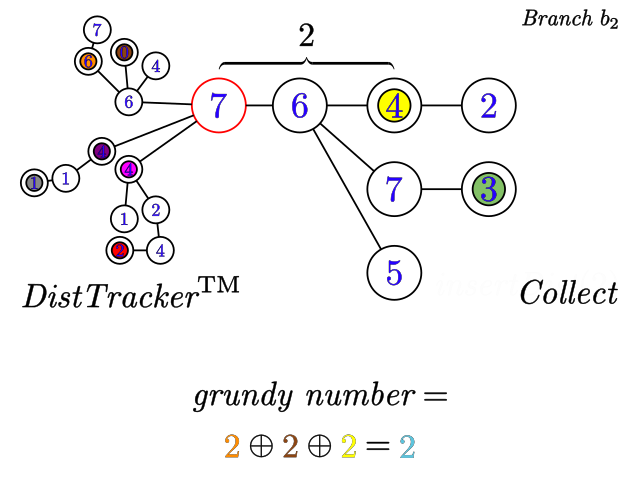Then we $\textit{clear}()$ our $\textit{DistTracker}$ and repeat, this time with $i=M,M-1,\dots,2,1$.

TODO - animation - MOVCOIN2_ED_5_THUMB.png, linking to MOVCOIN2_ED_5_ANIM.gif

We now return to optimising our $\textit{DistTracker}$. It often helps to take a bitwise approach to problems involving xor sums, and this is the case here. Let's have a look at the binary representation of an increasing series of numbers and observe how each bit changes. The numbers along the top of the table are the bit number with bit number $0$ being the least significant bit.

N 5 4 3 2 1 0
0 0 0 0 0 0 0
1 0 0 0 0 0 1
2 0 0 0 0 1 0
3 0 0 0 0 1 1
4 0 0 0 1 0 0
5 0 0 0 1 0 1
6 0 0 0 1 1 0
7 0 0 0 1 1 1
8 0 0 1 0 0 0

The pattern is clear: the $x^{\text{th}}$ bit is $0$ $2^x$ times in a row, then $1$ $2^x$ times in a row, and continues flipping every $2^x$ increments. We can exploit this pattern in our $\textit{DistTracker}$ to maintain a count of the number of tracked distances that have their $x^{\text{th}}$ bit set: the animation below illustrates this approach with the original example. Here:

• each tracked distance is represented by copies of the coin that led to that distance being inserted, one copy for each row (bit)
• scrolling the coins to the right represents incrementing the corresponding tracked distances
• the $x^{\text{th}}$ bit of a tracked distance is one if and only if its corresponding coin is in a red zone (called "the red one zone") for the $x^{\text{th}}$ row, as a consequence of the pattern above.

Note that the $x^{\text{th}}$ bit of the grundy number is set if and only if the number of tracked distances with their $x^{\text{th}}$ bit set is odd, so pairs of distances with their $x^{\text{th}}$ bit set contribute nothing to the grundy number and so are crossed out. If we know which bits of the grundy number are set, computing the number itself is trivial.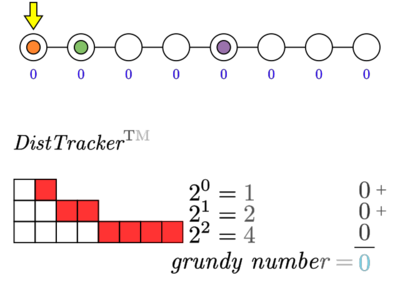Note that the fourth row of the grid is omitted: since the graph only has 8 nodes, the max distance between two nodes is seven, and so a tracked distance can never enter the red-one-zone for a fourth row and change the grundy number. Similar logic is used to reduce $\textit{m\_numBits}$ in the $\textit{DistTracker}$ implementation. In general, the number of bits/ rows is $\mathcal{O}(\log (\textit{max distance between nodes}))$.

With this new $\textit{DistTracker}$, the computation of the grundy number (the xor of all the tracked distances) has been rolled into $\textit{addToAllDists()}$, so $\textit{grundyNumber}()$ has been reduced from $\mathcal{O}(N)$ to $\mathcal{O}(1)$, a substantial improvement; however, $\textit{addToAllDists}()$ remains $\mathcal{O}(N)$ as it must still move all coins on each call, so we don't appear to have gained much.

However, what if on each call to $\textit{addToAllDists}(1)$, for each $x$, instead of moving all coins on row $x$ one cell to the right on and tracking whether they enter the red-one-zone, we scrolled the red-one-zone on that row by one to the left and counted how many coins its hits or leave? Since the number of rows is $\mathcal{O}(\log N)$, $\textit{addToAllDists}(1)$ is now $\mathcal{O}(\log N)$, so all operations on $\textit{DistTracker}$ are now $\mathcal{O}(\log N)$ in the worst case.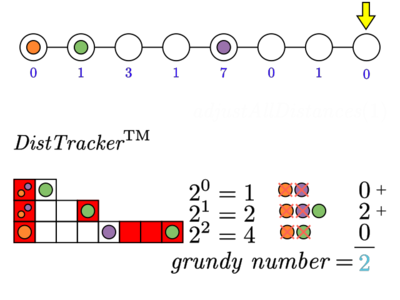And that's it!

TODO - end less lamely. Should maybe mention somewhere that reducing m_numBits gives asymptotic gains i.e. without it, creating a $\textit{DistTracker}$ for each Centroid $T_i$ would contribute $O(N^2)$ to the runtime

Complexity Analysis

• The $\textit{DistTracker}$ functions $\textit{insertDist}$, $\textit{addToAllDists}$ are $\mathcal{O}(\log N)$; $\textit{grundyNumber}$ is $\mathcal{O}(1)$. As an implementation note, my $\textit{DistTracker}$ does not have a $\textit{clear}$; I instead create a new $\textit{DistTracker}$ each time I need one (twice for each $T_i$ ‒ one for each direction), which is $\mathcal{O}(|T_i|)$ and so from C2 is $\mathcal{O}(N \log N)$ in total.
• There are are $\mathcal{O}(1)$ calls to each of $\textit{insertDist}$ and $\textit{addToAllDists}$ for each node in each $T_i$, so again from the above and C2, this is $\mathcal{O}(N \log N \log N)$ in total.

# ALTERNATE EXPLANATION:

A Faster Alternative to Centroid Decomposition? When I first solved this Problem (and CHGORAM2), I didn't know much about the properties of Centroid Decomposition and so came up with my own approach which I called the light-first DFS:

This runs quite a bit faster than my Centroid Decomposition based solution. Questions for the interested reader :)

• Why does this work? What is its worst-case complexity? How would you prove it?
• Are there any Problems for which a CD approach would work but not a LFDFS? Vice-versa?
• Is the speedup due to mere implementation details, or might there be certain trees where LFDFS is asymptotically better than CD? If yes, what kind of trees would you expect to be faster in practice?

The fastest solution that I saw was @sg1729's, running in just 0.63s.

# SOLUTIONS:

Setter's Solution (C++) https://www.codechef.com/viewsolution/37919044

Tester's Solution (Kotlin)

package MOVCOIN2

class Movcoin2Solver(private val N: Int, private val gph: List<List<Int>>, private val hasToken: List<Boolean>) {
private class XorOfElementPlusConstant (elements: List<Int>, val constantMax: Int) {
private val MAX_BITS = 17

val xored = IntArray(constantMax+1)

init {
for(b in 0 until MAX_BITS) {
var l = (1 shl b)
var r = (1 shl (b+1)) - 1

var cnt = 0

val freq = IntArray(constantMax+1)
for(it in elements) {
val target = it and ((1 shl (b+1)) - 1)
freq[target] = (freq[target] ?: 0) + 1
if (target in l..r) cnt++
}

for (d in 0..constantMax) {
if (cnt % 2 == 1) xored[d] += 1 shl b

cnt -= freq.getOrElse(r) { 0 }

l--
if(l < 0) l = (1 shl (b+1)) - 1
r--
if(r < 0) r = (1 shl (b+1)) - 1

cnt += freq.getOrElse(l) { 0 }
}
}
}

fun getXorOfElementsPlus(constant: Int) = xored[constant]
}

private val marked = BooleanArray(N)

private val subtreeSize = IntArray(N)
private val getMaxSubtreeSizeWhenUIsRemoved = IntArray(N)

private fun getCentroidInComponentOf(root: Int): Int {
val queue: java.util.Queue<Pair<Int,Int>> = java.util.ArrayDeque<Pair<Int,Int>>()
val order = mutableListOf<Pair<Int,Int>>()

while(!queue.isEmpty()) {
val (u, p) = queue.poll()
subtreeSize[u] = 1
getMaxSubtreeSizeWhenUIsRemoved[u] = 0
for(v in gph[u]) if(!marked[v] && v != p) queue.add(Pair(v, u))
}

order.reverse()

for((u, p) in order) {
if(p >= 0) subtreeSize[p] += subtreeSize[u]
}

val numNodes = subtreeSize[root]

for((u, p) in order) {
getMaxSubtreeSizeWhenUIsRemoved[u] = maxOf(getMaxSubtreeSizeWhenUIsRemoved[u], numNodes - subtreeSize[u])
if (p >= 0) {
getMaxSubtreeSizeWhenUIsRemoved[p] = maxOf(getMaxSubtreeSizeWhenUIsRemoved[p], subtreeSize[u])
}
if (getMaxSubtreeSizeWhenUIsRemoved[u] <= numNodes / 2) {
return u
}
}

return -1
}

private fun getGrundys(): List<Int> {
val grundy = IntArray(N)

fun process(root: Int, initialD: Int) {
val order = mutableListOf<Pair<Int,Int>>()

val queue: java.util.Queue<Triple<Int,Int,Int>> = java.util.ArrayDeque<Triple<Int,Int,Int>>()

while(!queue.isEmpty()) {
val (u, p, d) = queue.poll()
for(v in gph[u]) if(!marked[v] && v != p) queue.add(Triple(v, u, d+1))
}

val distances = mutableListOf<Int>()
for((u, d) in order) if(hasToken[u]) distances.add(d)

val maxDistance = order.maxBy(Pair<Int,Int>::second)!!.second

val ds = XorOfElementPlusConstant(distances, maxDistance)
for((u, d) in order) grundy[u] = grundy[u] xor ds.getXorOfElementsPlus(d)
}

val queue: java.util.Queue<Int> = java.util.ArrayDeque<Int>()

process(0, 1)

while(!queue.isEmpty()) {
val q = queue.poll()

process(q, 1)

val u = getCentroidInComponentOf(q)
marked[u] = true
process(u, 0)

}

//println(grundy.toList())
return grundy.toList()
}

private fun getAnswer(grundy: List<Int>): Long {
val MOD = 1000000007
var pow2 = 1
var ans = 0L
for(value in grundy) {
pow2 *= 2
pow2 %= MOD
if(value == 0) ans += pow2
}
return ans % MOD
}

}

class Movcoin2Connector(private val br: java.io.BufferedReader, private val bw: java.io.BufferedWriter) {
var sumN = 0

fun checkConstraints() {
require(sumN <= 200000)
}

fun run() {
require(N in 1..200000)

val grp = IntArray(N) { it }
fun getGroup(x: Int): Int{
val parents = generateSequence(x, { grp[it] }).takeWhile { grp[it] != it }
val r = grp[parents.lastOrNull() ?: x]
parents.forEach{ grp[it] = r }
return r
}

val gph = List(N) { mutableListOf<Int>() }
repeat(N-1) {
val (a, b) = br.readLine()!!.split(' ').map{ it.toInt() - 1 }

val p = getGroup(a)
val q = getGroup(b)
require(p != q)
grp[p] = q
}

require(C.all{ it in 0..16 })

val solver = Movcoin2Solver(N, gph, C.map{ it % 2 == 1 })
bw.write("\${solver.run()}\n")
}
}

fun main (args: Array<String>) {
val br = java.io.BufferedReader(java.io.InputStreamReader(System.in))
val bw = java.io.BufferedWriter(java.io.OutputStreamWriter(System.out))

}